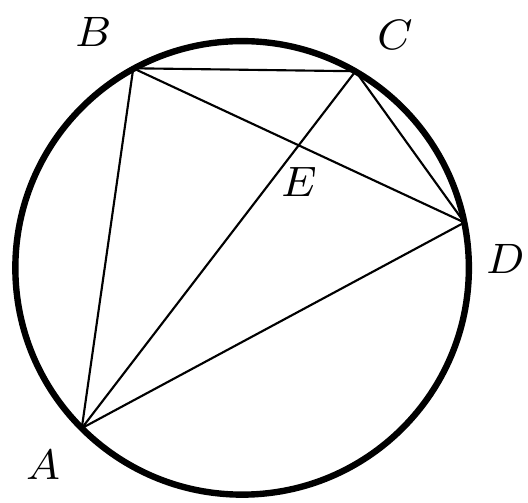Concyclic AreaMethod LawOfCosines PlaneGeometry Intermediate
1996

Problem - 3137

Let quadrilateral $ABCD$ inscribe a circle. If $BE=ED$, prove $$AB^2+BC^2 +CD^2 + DA^2 = 2AC^2$$The solution for this problem is available for $0.99. You can also purchase a pass for all available solutions for$99.